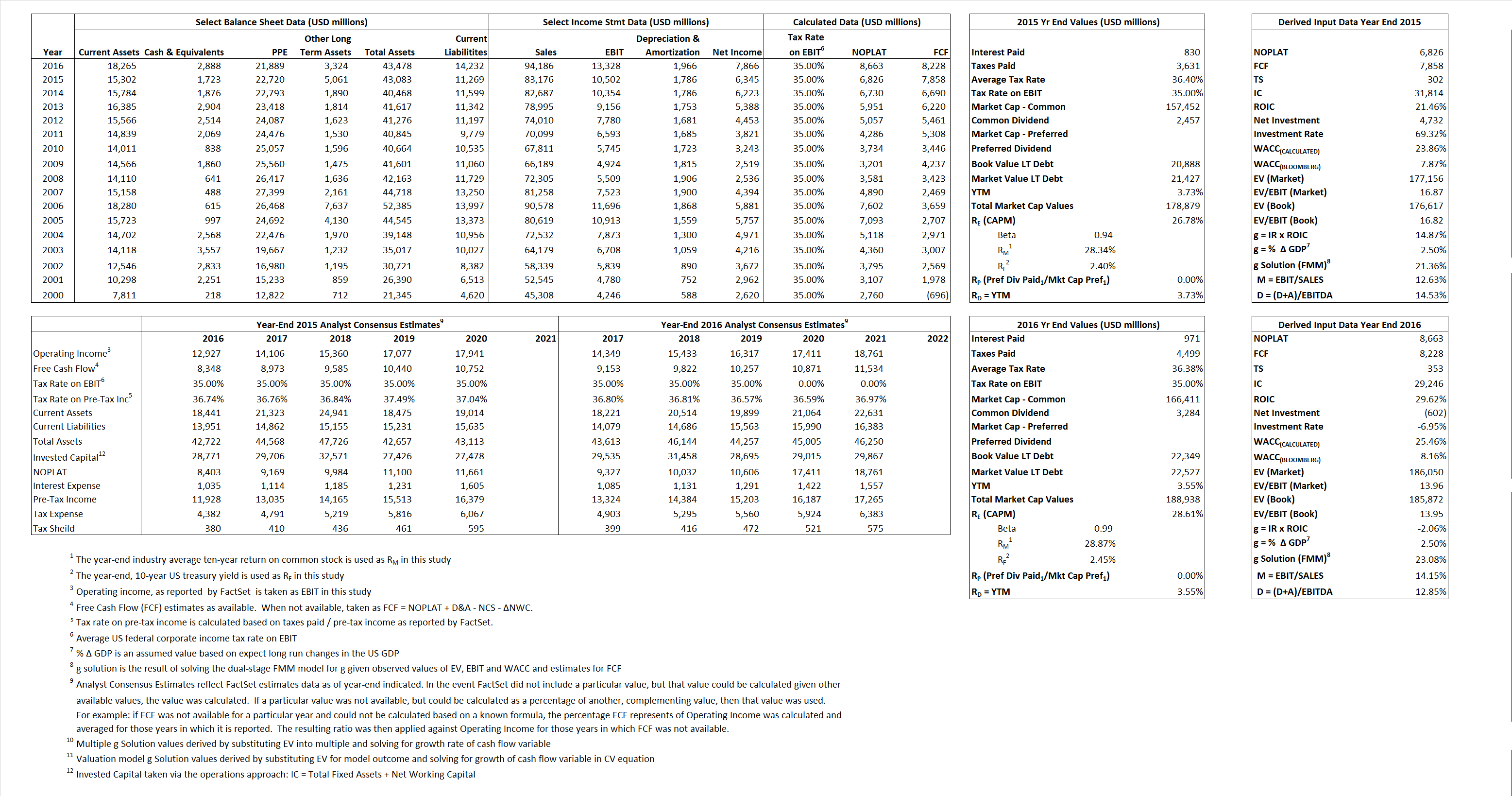# Home Depot, Inc.

## Analyst Listing

The following analysts provide coverage for the subject firm as of May 2016:

 Broker Analyst Analyst Email Longbow Research David S. MacGregor dmacgregor@longbowresearch.com Evercore ISI Greg Melich greg.melich@evercoreisi.com RBC Capital Markets Scot Ciccarelli scot.ciccarelli@rbccm.com Deutsche Bank Research Mike Baker michael.baker@db.com Telsey Advisory Group Joseph Feldman jfeldman@telseygroup.com Piper Jaffray Peter J. Keith peter.j.keith@pjc.com Nomura Research Jessica A. Schoen jessica.schoen@nomura.com Consumer Edge Research David A. Schick dschick@consumeredgeresearch.com Atlantic Equities Sam Hudson s.hudson@atlantic-equities.com Credit Suisse Seth Sigman seth.sigman@credit-suisse.com SunTrust Robinson Humphrey Keith Hughes keith.hughes@suntrust.com Oppenheimer Brian Nagel brian.nagel@opco.com Wolfe Research Scott Mushkin smushkin@wolferesearch.com Daiwa Securities Co. Ltd. Kahori Tamada kahori.tamada@us.daiwacm.com Wedbush Securities Seth Basham seth.basham@wedbush.com Jefferies Daniel Binder dbinder@jefferies.com Raymond James Budd Bugatch budd.bugatch@raymondjames.com BMO Capital Markets Wayne Hood wayne.hood@bmo.com

## Primary Input Data## Derived Input Data

### Equational Form

Net Operating Profit Less Adjusted Taxes NOPLAT  6,826 8,663$NOPLAT\, =\, EBIT\, x\, (1 \,-\, Avg \,\,Tax\,\, Rate\,\, on\,\, EBIT)$
Free Cash Flow FCF 7,858 8,228$FCF\,=NOPLAT\,+\,Non-Cash\,Expenses-\Delta NWC\,-\,NCS$
Tax Shield TS 302 353$TS\,=\,Interest\,\,Paid\,\,x\,\, Avg \,\,Tax\,\,Rate\,\, on\,\, Pre-Tax\,\, Income$
Invested Capital IC 31,814 29,246$IC\,=\,Fixed\,\,Operating\,\,Assets\,\,+\,\,Net\,\, Working\,\, Capital$
Return on Invested Capital ROIC 21.46% 29.62%$ROIC\,=\,\frac { NOPLAT }{ IC }$
Net Investment NetInv 4,732  (602)$NetInv\,=\,{ {IC}_{1}}-{{IC}_{0}}+Depreciation$
Investment Rate IR 69.32% -6.95%$IR\,=\,\frac {NetInv}{NOPLAT}$
Weighted Average Cost of Capital
WACCMarket 23.86% 25.46%$WACC\,=\,\frac { E }{ V } { R }_{ E }\,+\,\frac { P }{ V } { R }_{ P }\,+\,\frac { D }{ V } { R }_{ D }\left( 1- Avg\,\, Tax\,\,Rate\,\,on\,\,Pre-Tax\,\,Income \right)$
WACCBook   7.87%  8.16%
Enterprise value
EVMarket 117,1156  186,050$EV\,=\,Market\,\,Cap\,\,Equity\,+\,\,Long\,\,Term\,\,Debt\,-\,Cash$
EVBook  176,617 185,872
Long-Run Growth
g = IR x ROIC
14.87%  -2.06% Long-run growth rates of the income variable are used in the Continuing Value portion of the valuation models.
g = %$\Delta$ GDP    2.50%    2.50%
Margin from Operations M  12.63%   14.15%$M\,\,=\,\,\frac{EBIT}{SALES}$
Depreciation/Amortization Rate D  14.53%  12.85%$D\,\,=\,\,\frac{D+A}{EBITDA}$

## Valuation Multiple Outcomes

The outcomes presented in this study are the result of original input data, derived data, and synthesized inputs.

### model g solution

12/31/2015 12/31/2016 12/31/2015 12/31/2016 12/31/2015 12/31/2016

EV/SALES$\frac {EV}{Sales} \,= \,\frac{ROIC\, -\, g}{ROIC\,(WACC\,-\,g)}\,(1\,-\,T)\,(M)$

2.13  1.98  24.39%  24.69%  24.05%  25.24%

EV/EBITDA$\frac {EV}{EBITDA} \,= \,\frac{ROIC\, -\, g}{ROIC\,(WACC\,-\,g)}\,(1\,-\,T)\,(1\,-\,D)$

14.42 12.16 24.39% 24.69% 24.05% 25.24%

EV/NOPLAT$\frac {EV}{NOPLAT} \,= \,\frac{ROIC\, -\, g}{ROIC\,(WACC\,-\,g)}$

25.95 21.48 24.39% 24.69% 24.05% 25.24%

EV/FCFOPS$\frac {EV}{FCF_{OPS}} \,= \,\frac{ROIC\, -\, g}{ROIC\,(WACC\,-\,g)}\,(1\,-\,T)$

22.54 22.61 24.39% 24.69% 24.05% 25.24%

EV/EBIT$\frac {EV}{EBIT} \,= \,\frac{ROIC\, -\, g}{ROIC\,(WACC\,-\,g)}\,(1\,-\,T)$

16.87 13.96 24.39% 24.69% 24.05% 25.24%

EV/IC$\frac {EV}{IC} \,= \,\frac{ROIC\, -\, g}{WACC\,-\,g}$

5.57 6.36 24.39% 24.69% 24.05% 25.24%